# RD Sharma Solutions - Chapter 22 - Mensuration - III (Part - 1), Class 8, Maths Notes | Study RD Sharma Solutions for Class 8 Mathematics - Class 8

## Class 8: RD Sharma Solutions - Chapter 22 - Mensuration - III (Part - 1), Class 8, Maths Notes | Study RD Sharma Solutions for Class 8 Mathematics - Class 8

The document RD Sharma Solutions - Chapter 22 - Mensuration - III (Part - 1), Class 8, Maths Notes | Study RD Sharma Solutions for Class 8 Mathematics - Class 8 is a part of the Class 8 Course RD Sharma Solutions for Class 8 Mathematics.
All you need of Class 8 at this link: Class 8

PAGE NO 22.10:

Question 1:

Find the curved surface area and total surface area of a cylinder, the diameter of whose base is 7 cm and height is 60 cm.

Let r and h be the radius and the height of the cylinder.

Given:r = 7/2 cm
h = 60 cm

Curved surface area of the cylinder  = 2π × r × h
= 2 × 22/7 × 7/2 × 60
= 22 × 60 = 1320 cm2
Total surface area of the cylinder = 2π × r × (r + h)
= 2 × 22/7 × 7/2 × (7/2 + 60) = 22 × 127/2 = 11 × 127 = 1397cm2

Question 2:

The curved surface area of a cylindrical road is 132 cm2. Find its length if the radius is 0.35 cm.

Consider h to be the height of the cylindrical rod.
Curved surface area = 132 cm2
We know:
Curved surface area = 2 × π × r × h
132 = 2 × 22/7 × 0.35 × h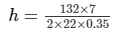h = 60
Therefore, the length of the cylindrical rod is 60 cm.

Question 3:

The area of the base of a right circular cylinder is 616 cm2 and its height is 2.5 cm. Find the curved surface area of the cylinder.

Given:Area of the base of a right circular cylinder =  616 cm2
Height =  2.5 cm
Let r be the radius of the base of a right circular cylinder.πr = 616
⇒ r= 616 × 7/22
⇒ r2 = 196
⇒ r = 14 cm
Curved surface area of the right circular cylinder = 2πrh = 2 × 22/7 × 14 × 2.5
=  220 cm

Question 4:

The circumference of the base of a cylinder is 88 cm and its height is 15 cm. Find its curved surface area and total surface area.

Given:Height, h = 15 cm
Circumference of the base of the cylinder = 88 cm2
Let r be the radius of the cylinder.The circumference of the base of the cylinder = 2πr
88 = 2 × 22/7 × r
r =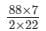= 14 cm
Curved surface area  = 2 × π × r × h
= 2 × 22/7 × 14 × 15
= 1320 cm2
Total surface area  = 2 × π × r × (r + h)
= 2 × 22/7 × 14 × (14 + 15)
= 2552 cm

Question 5:

A rectangular strip 25 cm × 7 cm is rotated about the longer side. Find The total surface area of the solid thus generated.

Since the rectangular strip of 25 cm × 7 cm is rotated about the longer side, we have:

Height, h = 25 cm

∴Total surface area  = 2πr(r + h) = 2π(7)(25 + 7)
= 14π(32) = 448πcm²
= 448 × 22/7cm²
= 1408 cm²

Question 6:

A rectangular sheet of paper, 44 cm × 20 cm, is rolled along its length to form a cylinder. Find the total surface area of the cylinder thus generated.

The rectangular sheet of paper 44 cm × 20 cm is rolled along its length to form a cylinder.

The height of the cylinder is 20 cm and circumference is 44 cm.
We have: Height, h = 20 cm
Circumference =  2πr = 44 cm
∴Total surface area is S = 2πrh = 44 × 20 cm²
= 880 cm²

Question 7:

The radii of two cylinders are in the ratio 2 : 3 and their heights are in the ratio 5 : 3. Calculate the ratio of their curved surface areas.

Let the radii of two cylinders be 2r and 3r, respectively, and their heights be 5h and 3h, respectively.Let S1 and Sbe the curved surface areas of the two cylinder.
S1 =  Curved surface area of the cylinder of height 5h and radius 2r
S2 =  Curved surface area of the cylinder of height 3h and radius 3r
∴ S1:S2 = 2 × π × r × h : 2 × π × r × h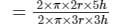= 10 : 9

Question 8:

The ratio between the curved surface area and the total surface area of a right circular cylinder is 1 : 2. Prove that its height and radius are equal.

Let S1 and S2 be the curved surface area and total surface area of the circular cylinder, respectively.

Then, S1 = 2πrh , S2 = 2πr(r + h)
According to the question:
S1:S2 = 1:2
2πrh : 2πr(r + h)  = 1 : 2
h : (r + h)  =  1 : 2
h/(r + h) = 1/2
2h = r + h
h = r
Therefore, the height and the radius are equal.

Question 9:

The curved surface area of a cylinder is 1320 cm2 and its base has diameter 21 cm. Find the height of the cylinder.

Let h be the height of the cylinder.
Given:Curved surface area, S = 1320 cm2
Diameter, d = 21 cm
S = 2πrh
1320 = 2π × 10.5 × h
h =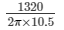h = 20 cm

PAGE NO 22.11:

Question 10:

The height of a right circular cylinder is 10.5 cm. If three times the sum of the areas of its two circular faces is twice the area of the curved surface area. Find the radius of its base.

Let r be the radius of the circular cylinder.Height, h =  10.5 cm
Area of the curved surface, S1 = 2πrh
Sum of the areas of its two circular faces, S2 = 2πr2
According to question:       3S= 2S1
3 × 2πr2 = 2 × 2πrh
6r = 4h
3r = 2h
r = 2/3 × 10.5 cm
= 7 cm

Question 11:

Find the cost of plastering the inner surface of a well at Rs 9.50 per m2, if it is 21 m deep and diameter of its top is 6 m.

Given:Height, h = 21 m
Diameter, d =  6 m
Area of the inner surface of the well, S = 2πrh
= 2π × 3 × 21 m2
=  2 × 22/7 × 3 × 21 m2 = 396 m2
According to question, the cost per m2 is Rs 9.50.
∴ Inner surface cost is Rs 396 × 9.50 = Rs 3762

Question 12:

A cylindrical vessel open at the top has diameter 20 cm and height 14 cm. Find the cost of tin-plating it on the inside at the rate of 50 paise per hundred square centimeter.

Given:Diameter, d = 20 cm
Height, h = 14 cm
Area inside the cylindrical vessel that is to be tin−plated = S
S = 2πrh + πr
= 2π × 10 × 14 + π × 10
= 280π + 100π
= 380 × 22/7 cm2
= 8360/7 cm2
According to question:Cost per 100 cm2  =  50 paise
Cost per cm2  =  Rs 0.005
Cost of tin−plating the area inside the cylindrical vessel =  Rs 0.005 × 8360/7 = Rs 41.8/7 = Rs 5.97

Question 13:

The inner diameter of a circular well is 3.5 m. It is 10 m deep. Find the cost of plastering its inner curved surface at Rs 4 per square metre.

Given:Inner diameter of the circular well  = 3.5 m
∴ Inner radius of the circular well, r = 1.75 m
Depth of the circular well, h = 10 m
Inner curved surface area, S = 2πrh
S = 2π × 1.75 × 10 m2 = 2 × 22/7 × 1.75 × 10 m2    = 110 m2
Cost of plastering 1 marea  = Rs 4
Cost of plastering 110 m2 area  = Rs (110 × 4) = Rs 440

Question 14:

The diameter of a roller is 84 cm and its length is 120 cm. It takes 500 complete revolutions moving once over to level a playground. What is the area of the playground?

Given:Diameter of the roller  = 84 cm
∴ Radius, r = Diameter/2 = 42 cm
In 1 revolution, it covers the distance of its lateral surface area.Roller is a cylinder of height,  h =  120 cm
Lateral surface area of the cylinder = 2πrh = 2 × 22/7 × 42 × 120 = 31680 cm2
It takes 500 complete revolutions to level a playground.
∴ Area of the field  = 31680 × 500 = 15840000 cm2                       (1 cm2 = 1/10000 m2)
∴ 15840000 cm2 = 1584 m2.
Thus, the area of the field in m2 is 1584 m2

Question 15:

Twenty one cylindrical pillars of the Parliament House are to be cleaned. If the diameter of each pillar is 0.50 m and height is 4 m, what will be the cost of cleaning them at the rate of Rs 2.50 per square metre?

Given:Diameter of the pillars = 0.5 m
Radius of the pillars, r = 0.25 m
Height of the pillars, h = 4 m
Number of pillars  = 21
Rate of cleaning  =  Rs 2.50 per square metre
Curved surface area of one pillar = 2πrh = 2 × 22/7 × 0.25 × 4 = 2 × 22/7 = 447 m2
∴ Curved surface area of one pillar = 44/7 m2
Cost of cleaning 21 pillars at the rate of Rs 2.50 per m2 =  Rs 2.5 × 21 × 44/7  = 7.5 × 44
∴ Cost of cleaning 21 pillars at the rate of Rs 2.50  per m2 =  Rs 330

Question 16:

The total surface area of a hollow cylinder which is open from both sides is 4620 sq. cm, area of base ring is 115.5 sq. cm and height 7 cm. Find the thickness of the cylinder.

Given:Total surface area of the cylinder = 4620 cm2
Area of the base ring =  115.5 cm2
Height, h = 7 cm. Let R be the radius of the outer ring and r be the radius of the inner ring.Area of the base ring  = πR2−πr2
115.5 = π(R2− r2)
R2− r2 = 115.5 × 7/22     (R + r)(R−r) = 36.75      ...........   (i)
Total surface area  =  Inner curved surface area  +  Outer curved surface area  +  Area of bottom and top rings
4620 = 2πrh + 2π
Rh + 2 × 115.52πh(R + r) = 4620−231
R + r =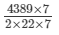R + r = 399/4        ...........   (ii)
Substituting the value of R + r from the equation (ii) in (i):(399/4)(R−r) = 36.75
(R−r) = 36.75 × 4/399 = 0.368 cm
∴ Thickness of the cylinder =  (R−r) = 0.368 cm

Question 17:

The sum of the radius of the base and height of a solid cylinder is 37 m. If the total surface area of the solid cylinder is 1628 m2, find the circumference of its base.

Let r and h be the radius and height of the solid cylinder.
Given:r + h = 37 m
Total surface area, S = 2πr(r + h)1628  = 2π × r × 37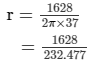= 7 m
Circumference of its base, S1 = 2πr     = (2 × 22/7 × 7)  m     = 44 m

Question 18:

Find the ratio between the total surface area of a cylinder to its curved surface area, given that its height and radius are 7.5 cm and 3.5 cm.

Let Sand Sbe the total surface area and curved surface area, respectively.
Given:Height, h = 7.5 cm
S1 = 2πr(r + h) S2 = 2πrh
According to the question: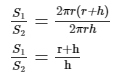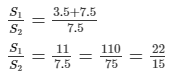Therefore, the ratio is 22:15.

Question 19:

A cylindrical vessel, without lid, has to be tin-coated on its both sides. If the radius of the base is 70 cm and its height is 1.4 m, calculate the cost of tin-coating at the rate of Rs 3.50 per 1000 cm2.

Let r cm and h cm be the radius of the cylindrical vessel.Given: Radius, r =  70 cm
Height, h = 1.4 m = 140 cm
Rate of tin−plating  =  Rs 3.50 per 1000 square centimetre

Cost of tin−plating the cylindrical vessel on both the surfaces (inner and outer):
Total suface area of a vessel =  Area of the inner and the outer side of the base  +  Area of the inner and the outer curved surface
= 2(πr2 + 2πrh)
= 2πr(r + 2h)
= 2 × 22/7 × 70 × (70 + 2 × 140)
= 44 × 10 × 350
= 154000 cm2
Cost of painting at the rate of Rs 3.50 per 1000 cm2 = 154000 × 3.50/1000 = Rs 539
Therefore, cost of painting is Rs 539.

The document RD Sharma Solutions - Chapter 22 - Mensuration - III (Part - 1), Class 8, Maths Notes | Study RD Sharma Solutions for Class 8 Mathematics - Class 8 is a part of the Class 8 Course RD Sharma Solutions for Class 8 Mathematics.
All you need of Class 8 at this link: Class 8Use Code STAYHOME200 and get INR 200 additional OFF

## RD Sharma Solutions for Class 8 Mathematics

88 docs

Track your progress, build streaks, highlight & save important lessons and more!

,

,

,

,

,

,

,

,

,

,

,

,

,

,

,

,

,

,

,

,

,

,

,

,

,

,

,

;